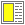# Exercise solution: Quantifier Functions

```; Directly programmed functions. These function terminate
; if the predicate fail on an element (for-all) or if
; if the predicate holds on an element (there-exists)
; Notice that both functions are tail-recursive.

(define (for-all lst p)
(cond ((null? lst) #t)
((p (car lst)) (for-all (cdr lst) p))
(else #f)))

(define (there-exists lst p)
(cond ((null? lst) #f)
((p (car lst)) #t)
(else (there-exists (cdr lst) p))))

; It is most natural to program there-exists-1 by use of
; filter - see below.

; Versions of for-all and there-exists programmed with
; mapping and accumulation. These function will traverse the
; whole list twice. This should be avoided, if possible.

(define (for-all lst p)
(accumulate-right and-fn #t (map p lst)))

(define (there-exist lst p)
(accumulate-right or-fn #f (map p lst)))

(define (there-exists-1 lst p)
(= (length (filter p lst)) 1))

```